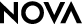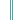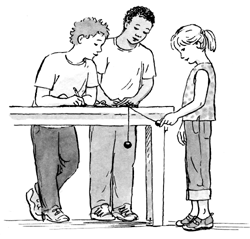Einstein's Big IdeaStudent Handout

Energy's Invisible World

You are probably familiar with the word energy. Besides the everyday use of the word to describe what you eat (energy foods, energy drinks) and how you feel (I had lots of energy, I completely ran out of energy), there are many other types of energy in the world around you. In this activity, you will examine different types of energy and how one type can be transferred into another. You will also observe how we know energy exists even though we cannot see it.Procedure

1. In this activity, you will move around the room in teams to different stations where you will make observations about energy and its transfer.

2. Choose one team member to record your team's observations.

3. Start at the station designated by your teacher and follow the directions on your "Station Instructions" handouts for each station.

4. When you have completed all the stations, answer the questions on this handout individually. Then discuss your answers as a team.

Questions

Write your answers on a separate sheet of paper.

1. At which station(s) did you observe the following field effects?

a) a magnetic field exerted a force on an object

b) an electric field applied an attractive force on an object

c) an electric field applied a repulsive force on an object

d) an electric field caused the formation of a magnetic field

2. At which station(s) did you observe the following energy transfers?

a) mechanical energy to heat energy

b) electrical energy to heat energy

c) potential energy to kinetic energy

d) mechanical energy to kinetic energy

e) kinetic energy to mechanical energy

f) chemical energy to heat energy

g) kinetic energy to sound energy

h) electrical energy to light energy

3. At which of the stations did you observe one kind of energy being converted to more than one other kind of energy? Draw a simple diagram to show the steps in the conversion of energy at each of these stations.

4. The law of conservation of energy says that energy cannot be created or destroyed; the total energy in a closed system remains constant (a system is a group of interrelated parts that function together as a whole). Design a procedure showing how you would test this law by modifying the open-system setups at Station 5, 6, or 7.

-->